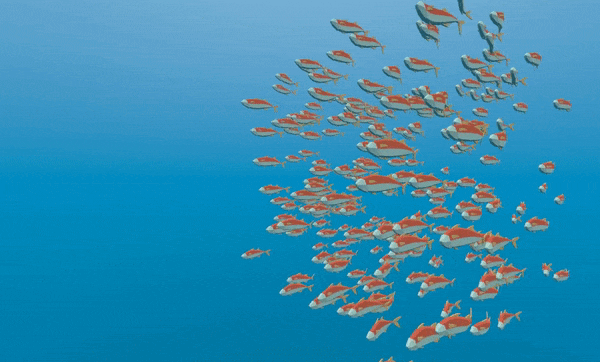# 用粒子控制数千条鱼¶

:参考:`网格实例<类_网格实例>`的问题是，更新转换数组的代价很高。它非常适合在场景周围放置许多静态对象。但在场景中移动物体，仍然很困难。

First create a Particles node. Then, under “Draw Passes” set the Particle’s “Draw Pass 1” to your Mesh. Then under “Process Material” create a new ShaderMaterial.

```shader_type particles
```

Then add the following two functions:

```float rand_from_seed(in uint seed) {
int k;
int s = int(seed);
if (s == 0)
s = 305420679;
k = s / 127773;
s = 16807 * (s - k * 127773) - 2836 * k;
if (s < 0)
s += 2147483647;
seed = uint(s);
return float(seed % uint(65536)) / 65535.0;
}

uint hash(uint x) {
x = ((x >> uint(16)) ^ x) * uint(73244475);
x = ((x >> uint(16)) ^ x) * uint(73244475);
x = (x >> uint(16)) ^ x;
return x;
}
```

First we will distinguish between code that needs to be run only when the particle system starts and code that should always run. We want to give each fish a random position and a random animation offset when the system is first run so we wrap that code in an `if` statement that checks the built-in variable `RESTART` which becomes `true` for one frame when the particle system is restarted.

```void vertex() {
if (RESTART) {
//Initialization code goes here
} else {
//per-frame code goes here
}
}
```

```uint alt_seed1 = hash(NUMBER + uint(1) + RANDOM_SEED);
uint alt_seed2 = hash(NUMBER + uint(27) + RANDOM_SEED);
uint alt_seed3 = hash(NUMBER + uint(43) + RANDOM_SEED);
uint alt_seed4 = hash(NUMBER + uint(111) + RANDOM_SEED);
```

```CUSTOM.x = rand_from_seed(alt_seed1);
vec3 position = vec3(rand_from_seed(alt_seed2) * 2.0 - 1.0,
rand_from_seed(alt_seed3) * 2.0 - 1.0,
rand_from_seed(alt_seed4) * 2.0 - 1.0);
```

```TRANSFORM.xyz = position * 20.0;
```

```VELOCITY.z = 10.0;
```

```VELOCITY.z = cos(TIME + CUSTOM.x * 6.28) * 4.0 + 6.0;
```

```CUSTOM.y = VELOCITY.z * 0.1;
```

This code gives you the following behavior: